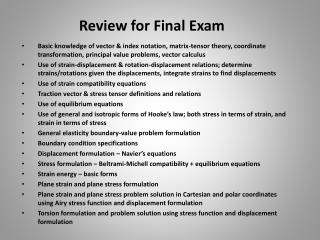DownloadDownload PresentationReview for Final Exam

# Review for Final Exam

Download Presentation## Review for Final Exam

- - - - - - - - - - - - - - - - - - - - - - - - - - - E N D - - - - - - - - - - - - - - - - - - - - - - - - - - -
##### Presentation Transcript

1. Review for Final Exam Basic knowledge of vector & index notation, matrix-tensor theory, coordinate transformation, principal value problems, vector calculus Use of strain-displacement & rotation-displacement relations; determine strains/rotations given the displacements, integrate strains to find displacements Use of strain compatibility equations Traction vector & stress tensor definitions and relations Use of equilibrium equations Use of general and isotropic forms of Hooke’s law; both stress in terms of strain, and strain in terms of stress General elasticity boundary-value problem formulation Boundary condition specifications Displacement formulation – Navier’s equations Stress formulation – Beltrami-Michell compatibility + equilibrium equations Strain energy – basic forms Plane strain and plane stress formulation Plane strain and plane stress problem solution in Cartesian and polar coordinates using Airy stress function and displacement formulation Torsion formulation and problem solution using stress function and displacement formulation